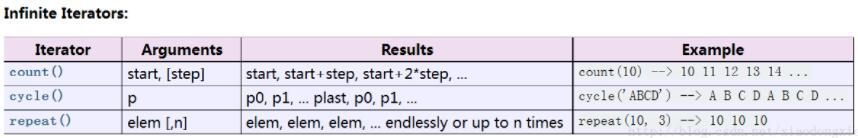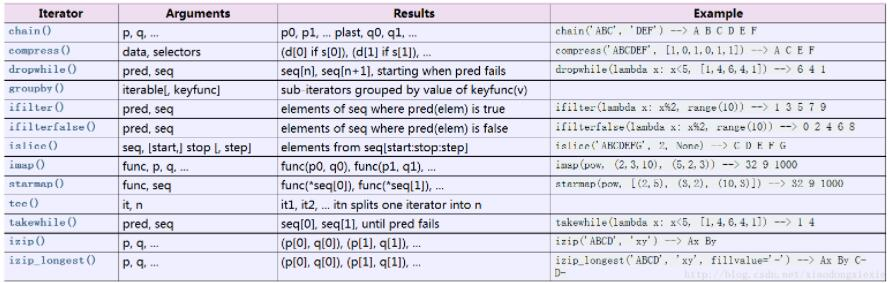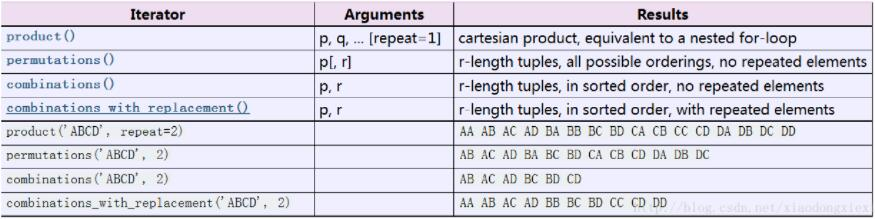# 對python中的高效迭代器函數詳解

更新時間：2018年10月18日 09:37:02   作者：曉東邪我要評論

python中內置的庫中有個itertools，可以滿足我們在編程中絕大多數需要迭代的場合，當然也可以自己造輪子，但是有現成的好用的輪子不妨也學習一下，看哪個用的順手~

```#import itertools
from itertools import * #最好使用時用上面那個，不過下面的是為了演示比較
常用的，所以就直接全部導入了```1、count（）

```for x in count(10,20):
if x < 200:
print x```
```def count(start=0, step=1):
# count(10) --> 10 11 12 13 14 ...
# count(2.5, 0.5) -> 2.5 3.0 3.5 ...
n = start
while True:
yield n
n += step```

2、cycle（）

```for i,x in enumerate(cycle('abcd')):
if i < 5:
print x```
```def cycle(iterable):
# cycle('ABCD') --> A B C D A B C D A B C D ...
saved = []
for element in iterable:
yield element
saved.append(element)
while saved:
for element in saved:
yield element```

3、repeat（）

```#注意如果不設置第二個參數notebook運行可能會宕機
for x in repeat(['a','b','c'],10):
print x```1、chain（）

```list(chain(range(4),range(5)))

list(chain(range(4),'abc'))

list(chain(('a','b','c'),'nihao',['shijie','zhongguo']))
```
```def chain(*iterables):
# chain('ABC', 'DEF') --> A B C D E F
for it in iterables:
for element in it:
yield element```

2.compress（）

`list(compress(['a','b','c','d','e'],[0,1,1,1,0,1]))`
```def compress(data, selectors):
# compress('ABCDEF', [1,0,1,0,1,1]) --> A C E F
return (d for d, s in izip(data, selectors) if s)```

3.dropwhile()

```#偽代碼大概是這個樣子的
if condition：
drop element
while not condition：
stop drop```
`list(dropwhile(lambda x:x>5,range(10,0,-1)))`
```def dropwhile(predicate, iterable):
# dropwhile(lambda x: x<5, [1,4,6,4,1]) --> 6 4 1
iterable = iter(iterable)
for x in iterable:
if not predicate(x):
yield x
break
for x in iterable:
yield x```

4.groupby

```for x,y in groupby(['a','a','b','b','b','b','c','d','e','e']):
print x
print list(y)
print ''

out:
a
['a', 'a']

b
['b', 'b', 'b', 'b']

c
['c']

d
['d']

e
['e', 'e']
```
```class groupby(object):
# [k for k, g in groupby('AAAABBBCCDAABBB')] --> A B C D A B
# [list(g) for k, g in groupby('AAAABBBCCD')] --> AAAA BBB CC D
def __init__(self, iterable, key=None):
if key is None:
key = lambda x: x
self.keyfunc = key
self.it = iter(iterable)
self.tgtkey = self.currkey = self.currvalue = object()
def __iter__(self):
return self
def next(self):
while self.currkey == self.tgtkey:
self.currvalue = next(self.it) # Exit on StopIteration
self.currkey = self.keyfunc(self.currvalue)
self.tgtkey = self.currkey
return (self.currkey, self._grouper(self.tgtkey))
def _grouper(self, tgtkey):
while self.currkey == tgtkey:
yield self.currvalue
self.currvalue = next(self.it) # Exit on StopIteration
self.currkey = self.keyfunc(self.currvalue)```

5.ifilter（）

```#這樣寫只是為了更清楚看到輸出，其實這么寫就跟filter用法一樣了，體現不到ifilter的優越之處了
list(ifilter(lambda x:x%2,range(10)))```

6.ifilterfalse（）

`list(ifilterfalse(lambda x:x%2,range(10)))`

7.islice()

```In: list(islice(range(10),2,None))
Out： [2, 3, 4, 5, 6, 7, 8, 9]

In: list(islice(range(10),2))
Out: [0, 1]
```

8.imap()

```#接受兩個參數時
list(imap(abs,range(-5,5)))

#接受三個參數時
list(imap(pow,range(-5,5),range(10)))

#接受四個參數時
list(imap(lambda x,y,z:x+y+z,range(10),range(10),range(10)))
```

9.starmap（）

```in： list(starmap(pow,[(1,2),(2,3)]))
out： [1, 8]```

10.tee()

```for x in list(tee('abcde',3)):
print list(x)```

11.takewhile()

```if condition：
take this element
while not condition：
stop take```

eg：

```in： list(takewhile(lambda x:x<10,(1,9,10,11,8)))
out： [1, 9]```

12.izip（）

`list(izip('ab','cd'))`

13.izip_longest

list(izip_longest('a','abcd'))1.product（）

```list(product(range(10),range(10)))

#本質上是這種的生成器模式
L = []
for x in range(10):
for y in range(10):
L.append((x,y))
```

2.permutations()

`list(permutations(range(10),2))`

3.combinations()

`list(combinations('abc',2))`
```def combinations(iterable, r):
# combinations('ABCD', 2) --> AB AC AD BC BD CD
# combinations(range(4), 3) --> 012 013 023 123
pool = tuple(iterable)
n = len(pool)
if r > n:
return
indices = range(r)
yield tuple(pool[i] for i in indices)
while True:
for i in reversed(range(r)):
if indices[i] != i + n - r:
break
else:
return
indices[i] += 1
for j in range(i+1, r):
indices[j] = indices[j-1] + 1
yield tuple(pool[i] for i in indices)```
```def combinations(iterable, r):
pool = tuple(iterable)
n = len(pool)
for indices in permutations(range(n), r):
if sorted(indices) == list(indices):
yield tuple(pool[i] for i in indices)```

4.combinations_with_replacement()

```def combinations_with_replacement(iterable, r):
# combinations_with_replacement('ABC', 2) --> AA AB AC BB BC CC
pool = tuple(iterable)
n = len(pool)
if not n and r:
return
indices =  * r
yield tuple(pool[i] for i in indices)
while True:
for i in reversed(range(r)):
if indices[i] != n - 1:
break
else:
return
indices[i:] = [indices[i] + 1] * (r - i)
yield tuple(pool[i] for i in indices)```
```def combinations_with_replacement(iterable, r):
pool = tuple(iterable)
n = len(pool)
for indices in product(range(n), repeat=r):
if sorted(indices) == list(indices):
yield tuple(pool[i] for i in indices)```